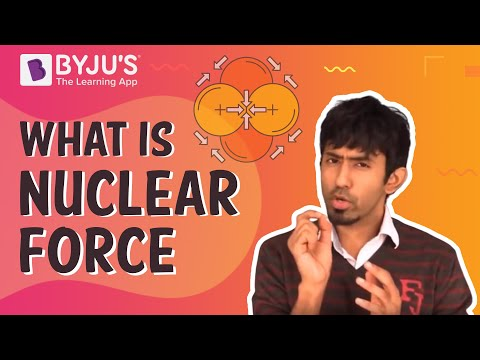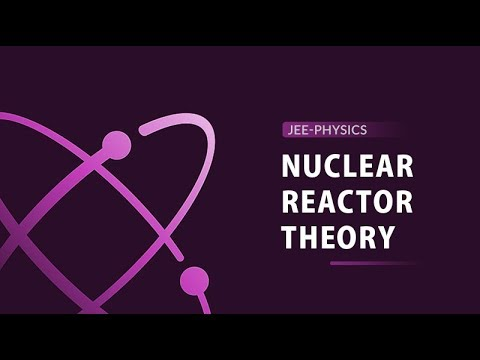Jet Set Go! All about Aeroplanes Jet Set Go! All about Aeroplanes

# Nuclear Energy Questions

A simultaneous release of energy occurs during nuclear changes. What is the source of this energy? If we could precisely measure the masses of the reactants and products of a nuclear reaction, we would discover that the quantity of mass transferred from reactants to products decreases slightly.

Nuclear reactions, either fission or fusion, release energy. Atoms unite to generate a larger atom in nuclear fusion. Atoms are divided to generate smaller atoms in nuclear fission, which releases energy. Nuclear reactors use nuclear fission to generate electricity. Nuclear fusion is the technique by which the Sun generates energy.

 Definition: The energy stored in the centre of an atom is known as nuclear energy. A tiny particle that makes up all matter in the universe is known as an atom. An atom’s mass is normally concentrated in the nucleus’s centre.

## Nuclear Energy Chemistry Questions with Solutions

Q1: Nuclear fusion is the phenomenon of ___________

a) Heavy nucleus splitting

b) Heavy nuclei combining

c) Light nucleus splitting

d) Light nuclei combining

Explanation: Nuclear fusion occurs when two or more lighter nuclei combine to form a single stable and heavy nucleus.

Q2: In which of the following processes are Neutrons emitted?

a) Nuclear fission

b) Inverse beta Decay

c) Spontaneous Fission

d) Nuclear fusion

Explanation: The splitting of a heavy nucleus into two or more lighter nuclei is known as nuclear fission. This results in a loss in mass, as well as exothermic energy and neutron emission. Fission elements are nuclei that emit two to three neutrons per nucleus.

Q3: In the Proton cycle, the hydrogen and deuteron fuse to form ___________

a) Deuteron

b) Helium – 3

c) Helium – 4

d) Tritium

Explanation: The process is as follows: 2D + 1H → 3He + Q X 2

The deuteron combines with a proton to yield Helium-3.

Q4: Why neutrons with lower energy should be capable of causing fission?

a) For sustained reaction process

b) For faster reaction process

c) For Safety purpose

d) In order to not waste the nuclear fuel

Answer: a) For sustained reaction process

Explanation: The initial high kinetic energy of a fission neutron is reduced due to collisions with other nuclei. Thus, even lower-energy neutrons should be able to cause fission in a long-term reaction. Only neutrons may cause a sustained reaction because each neutron absorbed by fission releases two or three neutrons.

Q5: What would be the effect of a nuclear holocaust?

a) Nuclear Summer

b) Nuclear Spring

c) Nuclear Winter

d) Nuclear Autumn

Explanation: The radioactive waste is expected to hang like a cloud in the earth’s atmosphere in the event of a nuclear holocaust. The sun’s radiation will be absorbed, resulting in a nuclear winter.

Q6: Mention the types of Nuclear Reactions.

Inelastic scattering: When energy is transferred, this phenomenon is called inelastic scattering. It occurs over the energy threshold. Et = ((A+1)/A)* ε1, where Et denotes the inelastic threshold energy and 1 denotes the energy of the first excited state.

Elastic Scattering: Elastic Scattering happens when energy is transferred from a particle to its intended nucleus. It is the most important method for slowing neutrons. The total kinetic energy of any system is conserved in the case of elastic scattering.

Transfer Reactions: Transfer reactions are defined as the absorption of one particle followed by the discharge of one or more particles.

Capture Reactions: Capture reactions happen when nuclei capture neutral or charged particles and then emit 𝝲-rays. Neutron capture processes let out radioactive nuclides.

Q7: How are nuclear reactions different from chemical reactions?

• Chemical reactions break and build bonds between two separate atoms, whereas nuclear reactions produce particles or rays from the nuclei of a single atom.
• Chemical reactions cause atomic rearrangements, although the atoms themselves stay unaltered. On the other hand, nuclear reactions involve a change in nuclear composition and hence a transformation of one type of atom into another.
• Nuclear reactions involve electrons, protons, and neutrons instead of valence electrons in chemical reactions.
• Small energy shifts occur in chemical processes. However, nuclear reactions result in significant energy changes.
• Temperature, reactant and product concentrations, pressure, and the presence of catalysts are all elements that influence the pace of a chemical reaction. Nuclear processes, on the other hand, are unaffected by any of these conditions, and their rates can range from milliseconds to millions of years.

Q8: Define nuclear transmutation. What causes nuclear transmutation?

The conversion of one chemical element or isotope into another chemical element is known as nuclear transmutation. Nuclear reaction or radioactive decay can both be used to create transmutation.

Transformations can occur as a result of nuclear decay of radioactive isotopes or neutron capture. Neither procedure requires a change in the atom’s element. An atom gets transmuted into an atom of a different element when the number of protons in it is changed.

The atomic number may remain unchanged after a neutron capture, but the quantity of nucleons is always increased. The isotope number changes, but the change is not chemical because the atom remains the same.

In unstable, radioactive elements, natural transmutation occurs when they decay into a series of other elements until a stable element is produced. As a result, uranium 238 spontaneously transmutes into stable lead 206.

Q9: Mention the factors affecting stability of Nucleus.

The following factors have an effect on nucleus stability:

1. Neutron-proton Ratio or n / p Ratio

It is the most important aspect in determining nucleus stability. The nuclei in the zone of stability, also known as the belt of stability, are stable. Above this zone, nuclei with a greater n/p ratio undergo β – emission to reach the stability zone.

1. Binding energy

When a nucleus is produced from its constituent nucleons, it releases energy. The higher the BE per nucleon, the more stable the nucleus. The maximum BE per nucleon for Fe is 8.8 MeV. As a result, it has the highest stability.

1. Packing fraction

It is the relative mass defect measurement.

Packing fraction = isotopic mass – mass no / mass no.*104

It can have a positive, negative, or zero value. Positive packing fraction nuclei are extremely unstable. Fe has the lowest packing fraction and H2 has the greatest.

1. Magic numbers

These numbers are referred to as magic numbers because nuclei with 2, 8, 20, 28, 50, 82, and 126 protons or neutrons are stable.

Even-numbered p+ and n0 nuclei are more stable than odd-numbered nuclei.

Q10: (i) Calculate the number of electrons which will together weigh one gram.

(ii) Calculate the mass and charge of one mole of electrons.

(i) Mass of an electron = 9.1 × 10−28 g

9.1 × 10−28 g is the mass of = 1 electron

∴ 1.0 g is the mass of =

$$\begin{array}{l}\frac{1}{9.1*10^{-28}} = 1.098 * 10^{27} electrons\end{array}$$

(ii) One mole of electrons = 6.022 × 1023 electrons

Mass of 1 electron = 9.1 × 10−31 kg

Mass of 6.022 × 1023 electrons = (9.1 × 10.31kg) × (6.022 × 1023) = 5.48 × 10−7 kg

Charge on one electron = 1.602 × 10−19 coulomb

∴ Charge on one mole electrons = 1.602 × 10−19 × 6.022 × 1023 = 9.65 × 104 coulombs

Q11: Mention the applications of Radioactivity.

1. Estimation of Age (Dating Technique)

(i) Carbon dating technique: It is used to evaluate wood, animal fossils, and other materials. It is based on the ratio of C14 to C12 in living species, which remains constant but drops in deceased samples. The age is calculated by comparing these two.

(ii) Uranium dating technique: Uranium-series dating, as the name implies, uses the radioactive decay of uranium to determine an age. When uranium decays, it undergoes a series of transformations before reaching a stable isotope. For instance, uranium 238 decays to uranium 234, which decays to thorium 230.

1. Medicinal Use

Many diseases are diagnosed and treated with radioisotopes. These can be utilised in three different ways: In Vivo studies, In therapeutic procedure (to cure diseases), and Imaging procedure.

Radioisotopes are also widely used in industry and agriculture to determine reaction mechanisms.

Q12: The half life period of a first order radioactive decay of an element is 5 days. What is the time (in days) taken for 8g of this element to decay to 1g?

The rate constant for a first-order reaction is calculated by using the following:

$$\begin{array}{l}k = \frac{0.693}{t_{(1/2)}}\end{array}$$
$$\begin{array}{l}k = \frac{0.693}{5} = 0.1386 day^{-1}\end{array}$$

Now, the formula for the time,

$$\begin{array}{l}t = \frac{2.303}{k} * log\frac{8}{1}\end{array}$$

t = 15 days

Radioactive disintegration is the process of converting one radioactive nucleus into another by the production of α, β and γ-rays.

Rutherford and Soddy proposed it in 1903.

γ-rays are secondary consequences of radioactive disintegration, meaning they are only emitted after α- and β-rays.

Methods of Radioactive Disintegration are as follows:

• Alpha (α) Decay: Emission of an α-particle decreases the atomic number by 2 units and mass number by 4 units.
• Beta (β) Decay: Emission of a β-particle increases the atomic number by 1 unit but does not affect the mass number.

Isotope is created through the emission of one α and two β-particles.

• Gamma (γ) Decay: The atomic number and mass number are not affected by the emission of γ-rays.

Q14: Technetium-99 is prepared from 98Mo. Molybdenum-98 combines with a neutron to give molybdenum-99, an unstable isotope that emits a β particle to yield an excited form of technetium-99, represented as 99Tc*. This excited nucleus relaxes to the ground state, represented as 99Tc, by emitting a γ ray. The ground state of 99Tc then emits a β-particle. Write the equations for each of these nuclear reactions.

$$\begin{array}{l}_{42}^{98}\textrm{Mo} + _{0}^{1}\textrm{n} \to _{42}^{99}\textrm{Mo}\end{array}$$
$$\begin{array}{l}_{42}^{99}\textrm{Mo} \to _{-1}^{0}\textrm{e} + _{43}^{99}\textrm{Tc}^{*}\end{array}$$
$$\begin{array}{l}_{43}^{99}\textrm{Tc}^{*} \to _{0}^{0}\textrm{v} + _{43}^{99}\textrm{Tc}\end{array}$$
$$\begin{array}{l}_{43}^{99}\textrm{Tc} \to _{-1}^{0}\textrm{e} + _{44}^{99}\textrm{Ru}\end{array}$$

Q15: Write a balanced equation for each of the following nuclear reactions:

1. the production of 17O from 14N by α particle bombardment
2. the production of 14C from 14N by neutron bombardment
3. the production of 233Th from 232Th by neutron bombardment
4. the production of 239U from 238U by 21H bombardment

1. $$\begin{array}{l}_{7}^{14}\textrm{N} + He^{2} \to _{8}^{17}\textrm{O} + _{1}^{1}\textrm{H}\end{array}$$
2. $$\begin{array}{l}_{7}^{14}\textrm{N} + _{0}^{1}\textrm{n} \to _{6}^{14}\textrm{C} + _{1}^{1}\textrm{H}\end{array}$$
3. $$\begin{array}{l}_{90}^{232}\textrm{Th} + _{0}^{1}\textrm{n} \to _{90}^{233}\textrm{Th}\end{array}$$
4. $$\begin{array}{l}_{92}^{238}\textrm{U} + _{1}^{2}\textrm{H} \to _{92}^{239}\textrm{U} + _{1}^{1}\textrm{H}\end{array}$$

## Practise Questions on Nuclear Energy

Q1: Who invented nuclear fission?

a) Rutherford

b) Otto Hahn

c) Hans Bethe

d) Marie Curie

Q2: Mention the applications of Nuclear Energy.

Q3: Define Nucleons and Nuclear Forces.

Q4: Define Accelerators.

Q5: Write in brief each of the following:

1. nucleon
2. α particle
3. β particle
4. positron
5. γ ray
6. nuclide

Click the PDF to check the answers for Practice Questions.

## Recommended Videos

#### Nuclear Force – Definition, properties, examples#### Theory of Nuclear Reactor